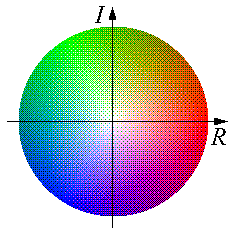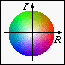# Introduction

Since we are dealing with amplitudes and phases, it is necessary two represent two values for each point in the pictures that follow. I have adopted a colourful scheme for representing complex numbers on the two-dimensional images. Amplitude is represented by colour saturation and brightness, while phase is given by hue. This is illustrated in the following figure:The positive real (horizontal) axis to the right represents a phase of 0. Phase increases anti-clockwise, thus 0 degrees is red, 120 degrees is green, and 240 degrees is blue.

Note that positive real numbers are red, and negative real numbers are cyan. White represents zero magnitude.Some Fourier transforms. Back to the index.